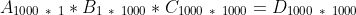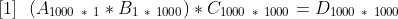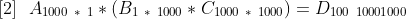A wrapper around numpy that does lazy evaluations to optimize for chained matrix multiplication

# lazynumpy

a lazy evaluated wrapper around numpy

What is gained?

• Chained matrix multiplication will be minimized by keeping the values of the other arrays in memory and solving the associative problem that minimizes the number of computations.
• only keeps one copy of each matrix [Memory optimization in progress]
• Allow partial matrix returns withou calculating the entire matrix [In Progress]

If you have three matrices with dimensions as below there are two ways to do the matrix multiplication to find the answer:Either:orwill take 1000 * 1 * 1000 operations to calculate A * B plus 1000 * 1000 * 1000 operations to calculate (A * B) * C. The total sum to calculate A * B * C is equal to 1000^3 + 1000^2.

 will take 1 * 1000 * 1000 operations to calculate B * C plus 1000 * 1 * 1000 operations to calculate A * (B * C). The total sum to calculate A * B * C is equal to 1000^2 + 1000^2 which means the optimal multiplication order will be ~500 faster.

If you run the simple example you should see a significant speed up. On my computer there is a 50x speedup with only three matrix calculations.

## Project details

This version0.0.1

Uploaded source
Uploaded py3